Next: FreeSurfaceFile: output Up: Input/Output files Previous: vertspace: input/output   Contents

### topology: input/output

Contains topology information per processor. (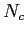= number of cells,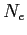= number of edges) Size: Depends on processor topology

• Type: ASCII
• Format: (int)Number of processors
• (int)Number of neighboring processors (Numneighs)
• (int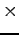Numneighs)Processor neighbor list
• For each neighboring processor: (int)Number of cells to send (cellsend)
• (int)Number of cells to receive (cellrecv)
• (int)Number of edges to send (edgesend)
• (int)Number of edges to receive (edgerecv)
• (intcellsend)Indices of cells to send
• (intcellrecv)Indices of cells to recv
• (intedgesend)Indices of edges to send
• (intedgerecv)Indices of edges to recv
• (int3)Indices for celldist array
• (int6)Indices for edgedist array
• (int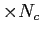)Indices for cellp array
• (int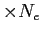)Indices for edgep array

2014-08-06